Friday, October 22, 2021
Home > CBSE Class 10 > Important MCQ Questions for Class 10 Maths Chapter 10 Circles

# Important MCQ Questions for Class 10 Maths Chapter 10 Circles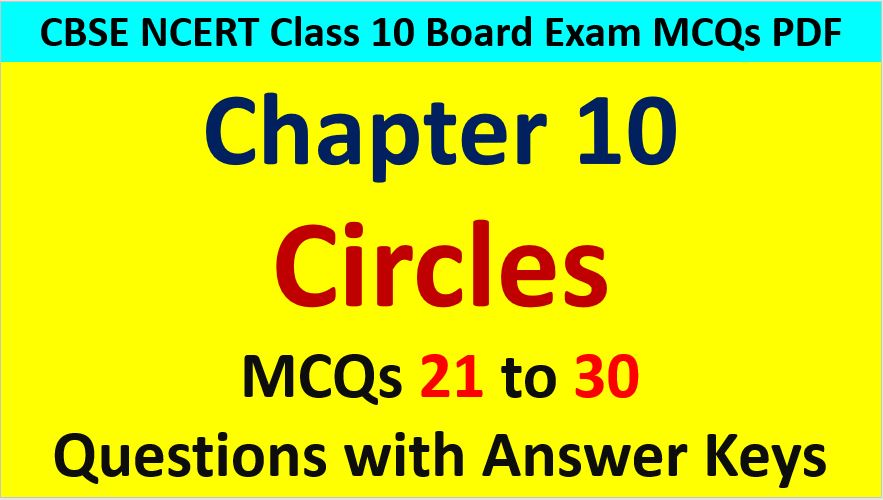Hi students, Welcome to AMBiPi (Amans Maths Blogs). In this article, you will get Important MCQ Questions for Class 10 Maths Chapter 10 Circles. You can download this PDF and save it in your mobile device or laptop etc.

Important MCQ Questions of Circles Class 10 Question No 21:

In the figure below, PT is tangent to the circle with centre O. Find the value of OT.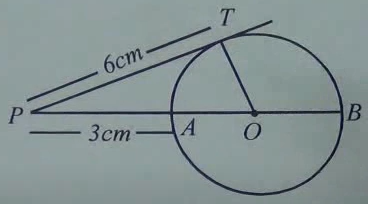Option A : 5.5 cm

Option B : 4.5 cm

Option C : 3.5 cm

Option D : 7 cm

Option B : 4.5 cm

Important MCQ Questions of Circles Class 10 Question No 22:

In the figure below, if PQ and PR are tangents to the circles with the centre O such that ∠QPR = 60o, then find the value of ∠QOR.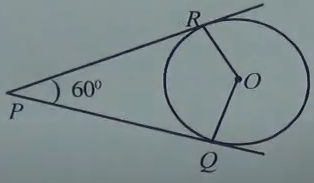Option A : 30o

Option B : 90o

Option C : 150o

Option D : 120o

Option D : 120o

Important MCQ Questions of Circles Class 10 Question No 23:

In the figure below, TS is a tangent to the circle with the center O such that ∠ABO = 32o, then find the value of x.Option A : 32

Option B : 58

Option C : 68

Option D : 48

Option B : 58

Important MCQ Questions of Circles Class 10 Question No 24:

In the figure below, PQ and PR are the tangents to a circle with center O such that ∠QOR = 110o, then the value of ∠OPR.Option A : 55

Option B : 70

Option C : 45

Option D : 35

Option D : 35

Important MCQ Questions of Circles Class 10 Question No 25:

In the figure below, circle with the O inscribed in the right angled triangle PQR, right angled at Q. If PQ = 5, QR = 12, then find the value of x.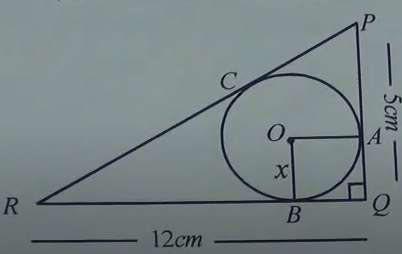Option A :

Option B :

Option C : 4

Option D : 1

Option B : 2

Important MCQ Questions of Circles Class 10 Question No 26:

In figure below, TX and TY are the tangents to a circle with center O. If ∠XOZ = 140o, then the value of ∠YXZ isOption A : 60

Option B : 30

Option C : 50

Option D : 40

Option A : 60

Important MCQ Questions of Circles Class 10 Question No 27:

In the figure below, if PA and PB are the tangents to a circle with the center O such that ∠APB = 80o, then the value of ∠OAB isOption A : 30

Option B : 40

Option C : 50

Option D : 80

Option B : 40

Important MCQ Questions of Circles Class 10 Question No 28:

In the figure below, if TA is a tangent to to the circle with center O and ∠ACB = 42o, then the value of ∠BAT isOption A : 48

Option B58

Option C : 42

Option D : 68

Option C : 42

Important MCQ Questions of Circles Class 10 Question No 29:

In the figure below, BOA is a diameter of a circle and the tangent at a point of P meets BA extended at T. If ∠PBO = 30 degrees, then the value of ∠PTA is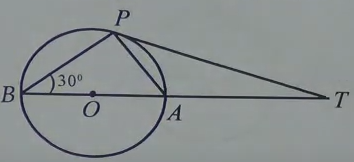Option A : 60

Option B : 30

Option C : 50

Option D : 40

Option B : 30

Important MCQ Questions of Circles Class 10 Question No 30:

In the figure below, if O is the centre of the circle and the tangent AC at point C makes an angle of 50 degree with chord BC, then find the value of ∠BOC.Option A : 120

Option B : 130

Option C : 100

Option D : 110